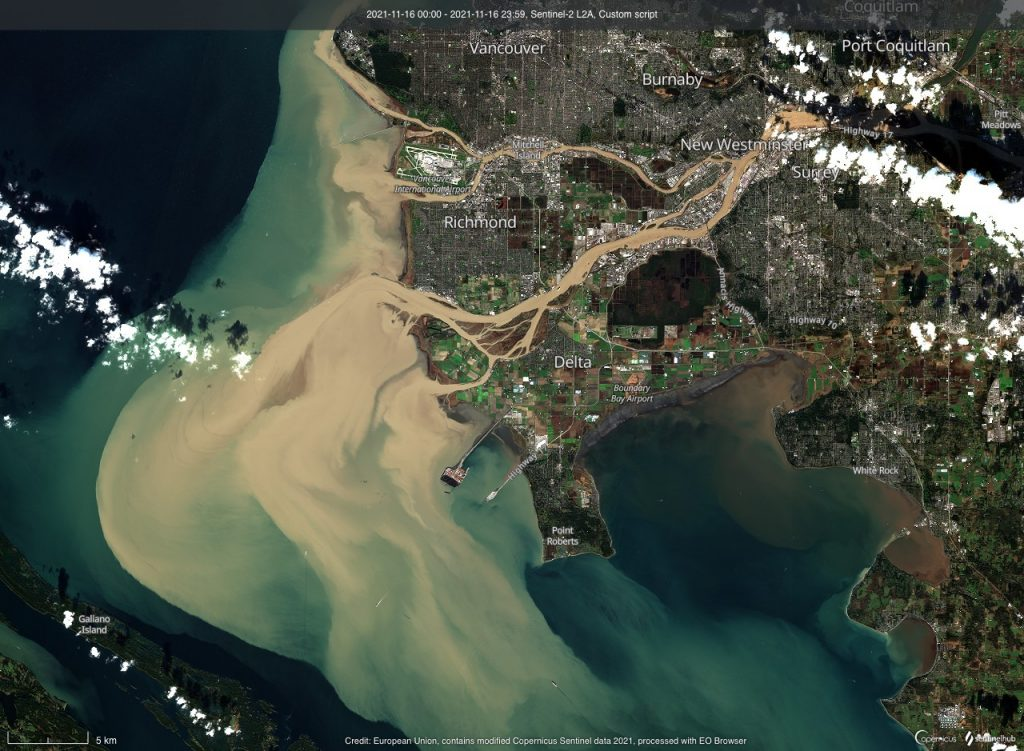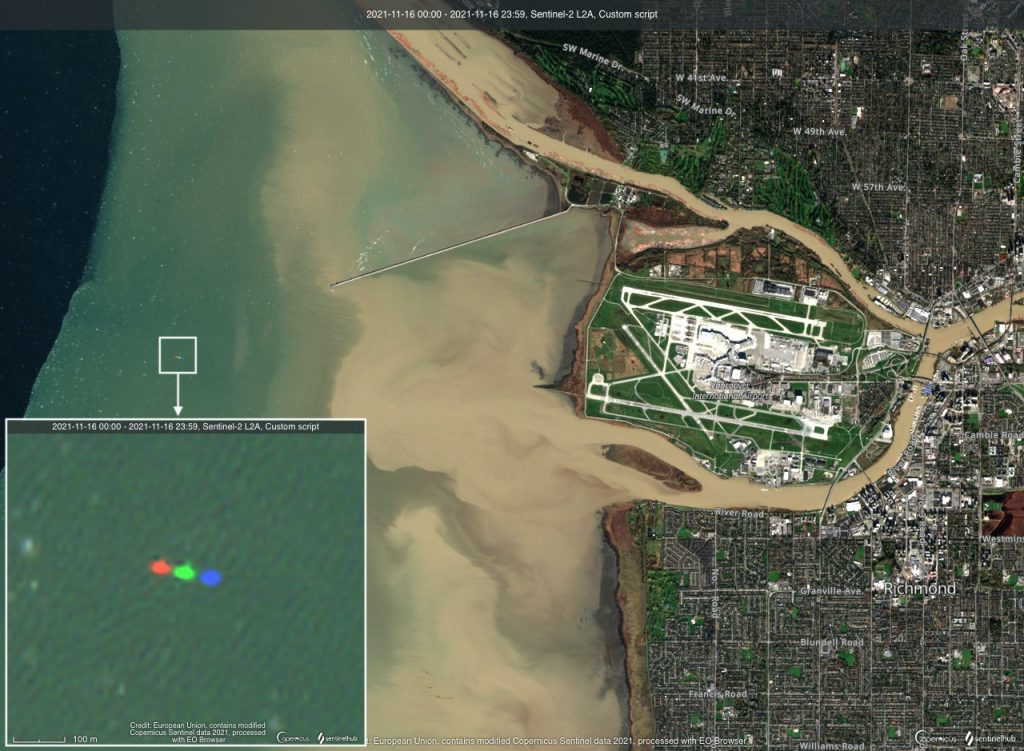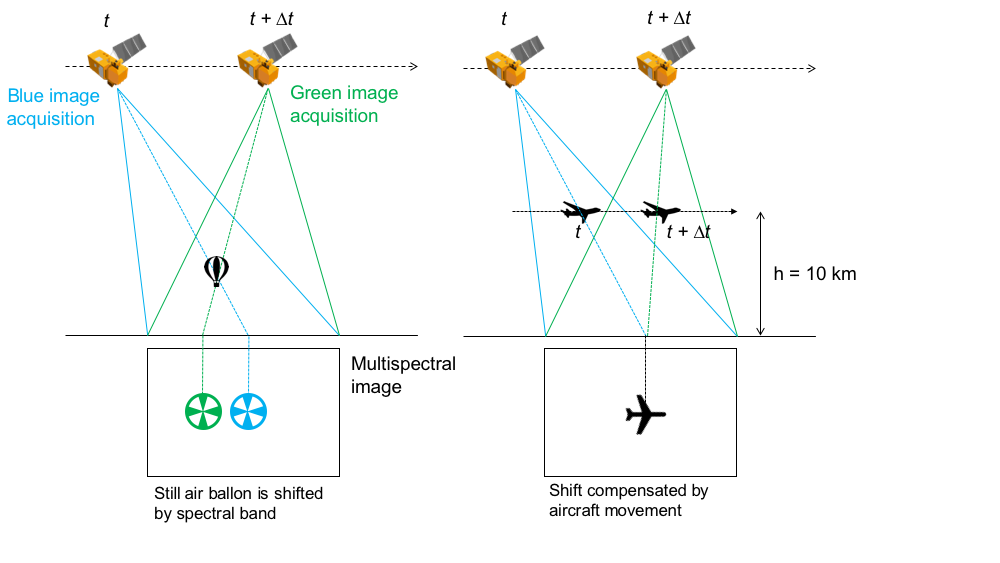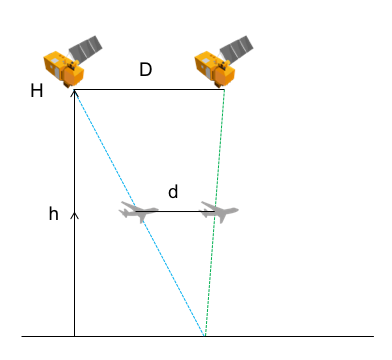Sentinel-2 captured an impressive view of the Fraser river sediment plume in Georgia Strait caused by the historic flood in British Columbia (250 mm rainfall in 48 hours).Fraser River delta and Georgia Strait near Vancouver, British Columbia, Canada. Sentinel-2 on 2021-Nov-16 (color composite of L2A red, greed and blue images).

The image contains an amusing detail west off the Vancouver International Airport. This red-green-blue object is an airplane or « plainbow« . It is due to shifts between the multi-spectral images composing the « true color » image (in this case an RGB image composed with the red, green and blue channels of a Sentinel-2 multispectral image).Misregistration shifts between multi-spectral images are well explained by Sergii Skakun et al. (2018):

The MSI is designed in such a way, that the sensor’s detectors for the different spectral bands are displaced from each other. This introduces a parallax angle between spectral bands that can result in along-track displacements of up to 17 km in the Sentinel-2A scene . Corresponding corrections using a numerical terrain model are performed to remove these interband displacements, so the MSI images, acquired in different spectral bands, are co-registered at the sub-pixel level to meet the requirement of 0.3 pixels at 99.7% confidence. However, these pre-processing routines cannot fully correct displacements for high altitude objects, e.g. clouds or fast moving objects such as airplanes or cars. Therefore, these types of objects appear displaced in images for different spectral bands. The magnitude of the displacement varies among pairs of bands depending on the inter-band parallax angle. For example, at 10 m spatial resolution, the maximum displacement is observed for bands 2 (blue) and 4 (red).

The parallax effect has been used to determine the elevation of a volcanic plume from Landsat imagery (de Michele et al. 2016). Here the object is an airplane, hence it is both moving at high velocity and it is not at the elevation of the digital terrain model at this location (i.e. sea level), therefore two different effects are contributing the color shift.Multispectral shift in the case of an air balloon floating above the surface (not moving) and an airplane flying at the right velocity and the right direction to compensate for this shift.

To better understand, let’s consider an airplane flying at an altitude of 10 km. Let’s imagine it is heading toward the same direction as Sentinel-2 (roughly from the north east to south west). What should be its velocity to compensate for the time delay between two spectral bands, so that both spectral images of the airplane are well aligned?The problem can be solved using Thales’ theorem.

$$\frac{d}{D}=\frac{h}{H}$$

where $$d$$ and $$D$$ are the distances traveled by the airplane and Sentinel-2 respectively during the time delay ∆t between two spectral band acquisitions:

$$d=v \Delta t$$

$$D=V \Delta t$$

where $$v$$ and $$V$$ are the velocities of the airplane and Sentinel-2 respectively.

Therefore we obtain:

$$v=V\frac{h}{H}$$

Sentinel-2 is flying at V = 7.44 km/s on its orbit at H = 786 km. Hence, at h = 10 km, we get v = 340 km/h. If the airplane was flying at 340 km/h towards the southwest it should not appear as a « plainbow » in a Sentinel-2 multispectral composite.

The same equation can be written in vector form to account for the airplane flying direction (see Eq. 7 in Heiselberg 2019). To determine the velocity and the elevation of an airplane from Sentinel-2 images, the system of two equations has three unknowns (velocity, altitude and heading angle) and cannot be solved without additional information or hypothesis. Heiselberg (2019) used airplane contrails to determine the airplane heading angle. However, the airplane elevation is very small with respect to Sentinel-2 altitude and most of the uncertainty actually comes from the low spatial resolution of Sentinel-2 images with respect to the airplane size. In the above case of the plainbow over Georgia Strait, I found a distance of $$d_{B2,B8A}$$ = 200 m ± 10 m between the airplane location in B2 and B8a (∆t = 2.055 s). Assuming that the airplane is at sea level (h = 0) we get v = 350 km/h ± 18 km/h, whereas we would get  v = 352 km/h at h = 1000 m. Hence, the error on the velocity due to the uncertainty on the distance estimate $$d_{B2,B8A}$$ is much larger than the uncertainty on the airplane elevation.

Ce site utilise Akismet pour réduire les indésirables. En savoir plus sur comment les données de vos commentaires sont utilisées.